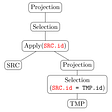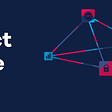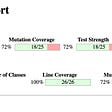# Overview

In this blog, we will understand what is a neon number and study the algorithm to write a java program that checks if the given number is neon number or not.

# What is Neon Number?

A neon number is a positive integer whose sum of digits of its square is equal to the number itself. There are only 3 neon numbers: 0, 1 and 9.

# Algorithm

1. Calculate the square of the given number.
2. Use a loop to find the sum of the digits of the square.
3. Return true if the sum is equal to the given number. Else, return false.

# How It Works

Here is the main logic to check if the given number is a neon number or not:

Let us input a number from the user and store it in a variable called “num”.

Find the square of the number and assign it to “square” (square = num * num).

Until the “square” remains as a positive integer, do the following steps:

1. Find the last digit of “square” and store it in a variable called “digit” (digit = square % 10). i.e. Divide the “square” with 10, the remainder gives the last digit of “square”.
2. Add the “digit” to a variable called “sum” which was initially assigned to 0.
3. As the last digit of “square” is added to “sum”, remove it form “square” (square = square / 10). i.e. Divide the “square” with 10, the quotient removes the last digit of “square”.

The “sum” variable contains the sum of the digits of square of the number.

Compare if the “sum” is same as the given number “num”.

Return true, if they match. Else, return false.

# Source Code

Here is the complete Java program that checks if the given number is Neon Number or not:

“Scanner” class and its function “next()” is used to obtain the input from the user and “println()” class is used to print the output. A static method “isNeon()” is called that checks for the neon number. It returns a boolean value ( true / false) which is stored in “result” variable. Then, the output is printed on the screen.

# Test Cases:

1.Enter a number: 18

18 is not a neon number.

2.Enter a number: 1

1 is a neon number.

--

--

--

Love podcasts or audiobooks? Learn on the go with our new app.

## Subquery Optimization in TiDB## Mainframe Product Update: September## IDE on the Cloud — AWS Cloud9## My favorite zsh plugins, 2020 edition## Best Practices To Follow While Creating Classes In Python## How To Fix Meizu 15 Plus Not Charging [Troubleshooting Guide]## What is Eclipse MicroProfile?## How to improve quality of the automated Java tests using Pitest